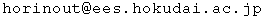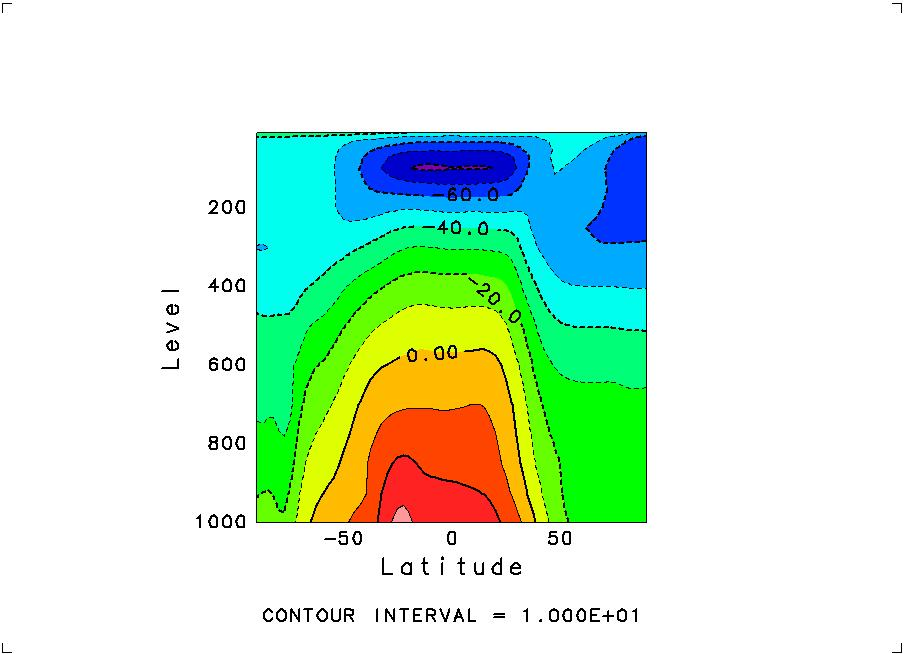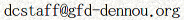Return to: NetCDFruby -- Ruby interface to NetCDF

# NetCDFruby tutorial

### demo0.1 (command line usage)

Ruby can be used interactively like a shell. If you have installed Ruby properly, a command named "irb", meaning "interactive ruby", has also been installed in your computer. To use it, simply type in
```% irb
```
except "%", which is meant to represent the prompt on your terminal.
Then the next prompt appears on your terminal to prompt your input to irb:
```irb(main):001:0>
```
If you input a line, the next prompt will be
```irb(main):002:0>
```

After installing NetCDFruby, cut and paste characters after prompts in the following. Lines that do not start with prompts are the outputs you will see. Italicized characters following "#" are comments attached afterwards for your information.

```irb(main):001:0> require "numru/netcdf"                    # load NetCDFruby
true
irb(main):002:0> file = NumRu::NetCDF.create("test.nc")     # create a file
NetCDF:test.nc
irb(main):003:0> dim = file.def_dim("x",3)           # create a dimension named "x" and length==3
NetCDFDim:x
irb(main):004:0> v=file.def_var("var","float",[dim]) # create a 1D variable with the 1st dim=="x"
NetCDFVar:test.nc?var
irb(main):005:0> file.close                          # close the file
nil
irb(main):006:0> print `ncdump test.nc`
netcdf test {
dimensions:
x = 3 ;
variables:
double var(x) ;                              # "float" for ruby is double in C. Use "sfloat" to define the float of C
data:

var = _, _, _ ;                                     # empty because values have not been set
}
nil
```

### demo0.2 (optional arguments)

Some arguments of some methods are optional, meaning default values are set if omitted. This feature is introduced here with an example.

Class method "open":
By default, a file is opened as read-only as with the predefined File class. Therefore,

```   file = NumRu::NetCDF.open("hogehoge.nc")
```
fails unless the file "hogehoge.nc" exists. The class method open takes two optional variables, mode and share, as its definition is
```   def NumRu::NetCDF.open(filename,mode="r",share=false)
```
A file is opened as writable if mode is set to "a" or "r+". The file is created if it is not found. Thus the method behaves like NetCDF.create in this case. The second optional argument is whether to use the NC_SHARE mode or not (see the NetCDF documentation for its meaning). The default is false as with the original NetCDF library. Follow the following examples to set these options:
```   file = NumRu::NetCDF.open("hogehoge.nc","a")          # writable, no share
file = NumRu::NetCDF.open("hogehoge.nc","a",true)     # writable, share
file = NumRu::NetCDF.open("hogehoge.nc","r",false)    # read-only, share
```
The IO mode follows the predefined File class of Ruby, which is based on the IO mode of the C language: "r" (read only), "w","w+" (write -- current contents are overwritten (eliminated!)), and "r+","a","a+" (append -- writable while current contents are preserved). Here, "r+" and "a" is the same, since NetCDF is not sequential. Also, you can always read in any mode, so "w" and "w+" are the same. The binary flag "b" is meaningless (thus "r" and "rb" is the same), since NetCDF is always binary. As seen above, to give the second argument is mandatory to specify the third one. Therefore, what seems to be less used is placed later (that is, we think the share option is the least used).

### demo1 (file creation)

The following program creates a file and prints its contents.

The contents of file demo1-create.rb:
(*NOTE*: The number at the beginning of each line in what follows is the line number attached afterwards for reference. It is NOT included in the source code. To download it, click here)

``` 1:  require "numru/netcdf"
2:  include NumRu
3:
4:  file = NetCDF.create("test.nc")
5:  nx, ny = 10, 5
6:  xdim = file.def_dim("x",nx)
7:  ydim = file.def_dim("y",ny)
8:  tdim = file.def_dim("t",0)
9:  require "date"
10:  file.put_att("history","created by #{\$0}  #{Date.today}")
11:
12:  x = file.def_var("x","sfloat",[xdim])
13:  y = file.def_var("y","sfloat",[ydim])
14:  t = file.def_var("t","sfloat",[tdim])
15:  v1 = file.def_var("v1","sfloat",[xdim,ydim])
16:  v1.put_att("long_name","test 1")
17:  v1.put_att("units","1")
18:  v2 = file.def_var("v2","sfloat",[xdim,ydim,tdim])
19:  v2.put_att("long_name","test 2")
20:  v2.put_att("units","1")
21:  file.enddef
22:
23:  x.put( NArray.float(nx).indgen! )
24:  y.put( NArray.float(ny).indgen! )
25:
26:  z = NArray.float(nx,ny).indgen!*0.1
27:  v1.put(z)
28:  v1.put( NArray.float(nx).add!(20), "start"=>[0,2],"end"=>[-1,2])
29:  v2.put(z, "start"=>[0,0,0],"end"=>[-1,-1,0])
30:  t.put( 0, "index"=>)
31:  v2.put(-z, "start"=>[0,0,1],"end"=>[-1,-1,1])
32:  t.put( 1, "index"=>)
33:
34:  file.close
35:  print `ncdump test.nc`
```
Here, Line 1 loads the NetCDF library.
Line 4-20 defines a netcdf file, and Line 21 ends the define mode to start the data mode to write values in variables.
Line 15 can also be written as
```v1 = file.def_var("v1","sfloat",["x","y"])
```
Therefore, the program can be rewritten alternatively as demo1-create-alt.rb.
Line 23-32 puts values in the variables, and Line 34 closes the file.
Line 35 prints the contents of the file just made. Note that `ncdump test.nc` executes the command "ncdump test.nc" and returns resultant standard output as a string, which is printed by the "print" global method.

To execute the program, type the following line (except for the prompt "%"):

```% ruby demo1-create.rb
```
Then, the following will be printed on your command-line terminal because of Line 35.
```netcdf test {
dimensions:
x = 10 ;
y = 5 ;
t = UNLIMITED ; // (2 currently)
variables:
float x(x) ;
float y(y) ;
float t(t) ;
float v1(y, x) ;
v1:long_name = "test 1" ;
v1:units = "1" ;
float v2(t, y, x) ;
v2:long_name = "test 2" ;
v2:units = "1" ;

// global attributes:
:history = "created by demo1-create.rb  2001-09-17" ;
data:

x = 0, 1, 2, 3, 4, 5, 6, 7, 8, 9 ;

y = 0, 1, 2, 3, 4 ;

t = 0, 1 ;

v1 =
0, 0.1, 0.2, 0.3, 0.4, 0.5, 0.6, 0.7, 0.8, 0.9,
1, 1.1, 1.2, 1.3, 1.4, 1.5, 1.6, 1.7, 1.8, 1.9,
20, 20, 20, 20, 20, 20, 20, 20, 20, 20,
3, 3.1, 3.2, 3.3, 3.4, 3.5, 3.6, 3.7, 3.8, 3.9,
4, 4.1, 4.2, 4.3, 4.4, 4.5, 4.6, 4.7, 4.8, 4.9 ;

v2 =
0, 0.1, 0.2, 0.3, 0.4, 0.5, 0.6, 0.7, 0.8, 0.9,
1, 1.1, 1.2, 1.3, 1.4, 1.5, 1.6, 1.7, 1.8, 1.9,
2, 2.1, 2.2, 2.3, 2.4, 2.5, 2.6, 2.7, 2.8, 2.9,
3, 3.1, 3.2, 3.3, 3.4, 3.5, 3.6, 3.7, 3.8, 3.9,
4, 4.1, 4.2, 4.3, 4.4, 4.5, 4.6, 4.7, 4.8, 4.9,
-0, -0.1, -0.2, -0.3, -0.4, -0.5, -0.6, -0.7, -0.8, -0.9,
-1, -1.1, -1.2, -1.3, -1.4, -1.5, -1.6, -1.7, -1.8, -1.9,
-2, -2.1, -2.2, -2.3, -2.4, -2.5, -2.6, -2.7, -2.8, -2.9,
-3, -3.1, -3.2, -3.3, -3.4, -3.5, -3.6, -3.7, -3.8, -3.9,
-4, -4.1, -4.2, -4.3, -4.4, -4.5, -4.6, -4.7, -4.8, -4.9 ;
}
```

### demo2 (simple visualization)

The program below (1) creates a file and close it. Then it (2) reopens the file and visualizes a variable in it. In the program, (1) and (2) are separated into two independent methods, write_file and draw_graph, respectively, just to clarify the separation; of course the program can be rewritten into only one main progam. Visualization is done with RubyDCL, so it must have been installed. Contents of demo2-graphic.rb:
``` 1:require "numru/dcl"
2:require "numru/netcdf"
3:include NumRu
4:include NMath
5:
6:## < create a sample netcdf file >
7:def write_file
8:
9:   file = NetCDF.create("test.nc")
10:   nx, ny = 21, 21
11:   xdim = file.def_dim("x",nx)
12:   ydim = file.def_dim("y",ny)
13:
14:   x = file.def_var("x","sfloat",["x"])
15:   y = file.def_var("y","sfloat",["y"])
16:   var = file.def_var("var","float",["x","y"])
17:   var.put_att("long_name","test variable")
18:   file.enddef
19:
20:   vx =  NArray.float(nx).indgen! * (2*(Math::PI)*1.5/(nx-1))
21:   vy =  NArray.float(ny).indgen! * (2*(Math::PI)*1.0/(ny-1))
22:   x.put( vx )
23:   y.put( vy )
24:
25:   sx = sin( vx )
26:   sy = sin( vy )
27:   z = NArray.float(nx,ny)
28:   for j in 0..ny-1
29:      z[true,j] = sx * sy[j] + 0.00001
30:   end
31:
32:   var.put(z)
33:
34:   file.close
35:   print `ncdump -h test.nc`
36:end
37:
38:## < read the file and plot >
39:def draw_graph
40:
41:   file = NetCDF.open("test.nc")
42:   vx = file.var("x")
43:   vy = file.var("y")
44:   vz = file.var("var")
45:   x = vx.get
46:   y = vy.get
47:   z = vz.get
48:   file.close
49:
50:   #DCL.swlset('ldump',1)
51:   DCL.gropn(1)
52:   DCL.grfrm
53:   DCL.usspnt(x, y)
54:   DCL.uspfit
55:   DCL.usdaxs
56:   DCL.sglset("lsoftf",false)
57:   DCL.uegtlb(z, -20)  # set the number of levels
58:   DCL.uelset("ltone",true)
59:   DCL.uetone(z)
60:   DCL.udcntz(z)
61:   DCL.grcls
62:end
63:
64:###(main)###
65:write_file
66:draw_graph
67:###(main)###
```
To execute the program, type the following line (except for the prompt "%"):
```% ruby demo2-graphic.rb
```
Then you will see the next image plotted to appear on your display.### demo3 (more elaborate visualization)

This is a more sophisticated (and thus longer as you would expect) example than the previous one. It plots arbitrary 2D slices of the 4D distribution of global temperature climatology from the NCEP reanalysis data. The data (8MB) is downloaded by anonymous ftp if it is not found in the user's run-time directory and he or she wants it.

Contents of demo3-ncepclim.rb:

```  1:# Plot global climatological temperature distribution
2:# from the NCEP reanalysis data.
4:
5:######################################
6:### local functions  ###
7:def nearest_index(na,val)
8:   # returns the element of na nearest to val
9:   # na is assumed to be 1d and monotonic
10:
11:   if(na > na[-1]) then
12:      reversed = true
13:      na = na[-1..0]
14:   else
15:      reversed = false
16:   end
17:
18:   w = (na.lt(val)).where
19:   idx = [ (w.length==0 ? 0 : w.max), na.length-2 ].min
20:   if ( na[idx+1]-val < val-na[idx] ) then
21:      idx = idx+1
22:   end
23:
24:   if reversed then
25:      na = na[-1..0]
26:      idx = na.length-1-idx
27:   end
28:
29:   idx
30:end
31:#####################################
32:### main part ###
33:require "numru/dcl"
34:require "numru/netcdf"
35:include NumRu
36:
37:filename = "air.mon.ltm.nc"
38:
40:
41:if !(Dir.glob(filename)) then
43:   host = "ftp.cdc.noaa.gov"
44:   path = "/Datasets/ncep.reanalysis.derived/pressure/"+filename
45:   print "\n*** question ***\n",
46:         "  File "+filename+" is not in the current directory.\n",
47:         "  Would you like to download (ftp) it from "+host+"?\n",
48:         "  (y, n)> "
49:   ans = gets
50:   if ans =~ /^y/ then
52:      email = gets.chop!
53:      require "net/ftp"
54:      print "  connecting...\n"
55:      ftp = Net::FTP.open(host, "anonymous", email, nil)
56:      size = ftp.size(path)
57:      print "  Size of the file is #{(size/1000)} kb. Would you really like to download?\n  (y, n)> "
58:      ans = gets
59:      if ans =~ /^y/ then
61:     ftp.getbinaryfile(path, filename)
62:      else
63:     print "  exit\n"
64:     exit
65:      end
66:   else
67:      print "  exit\n"
68:      exit
69:   end
70:end
71:
72:# < open the file and read axes >
73:
74:file = NetCDF.open(filename)
75:var = file.var("air")      # temperature
76:
77:lon = file.var("lon")
78:lat = file.var("lat")
79:level = file.var("level")
80:time = file.var("time")     # in hours
81:t = (time.get/720).round + 1     # --> in months
82:axes = [ lon, lat, level, time ]
83:axvals = [ lon.get, lat.get, level.get, t ]
84:axunits = [ lon.att("units").get.gsub("_",""),
85:            lat.att("units").get.gsub("_",""),
86:            level.att("units").get.gsub("_",""),
87:            "months" ]
88:# < graphics >
89:
90:#DCL.sglset('lbuff',false)
91:DCL.swlset('lwait',false)
92:DCL.gropn(1)
93:
94:first = true
95:while true do
96:   begin
97:
98:      ## / select a 2D slice /
99:
100:      if (first) then
101:     print <<-EOS
102:     ** select a slice **
103:     List desired grid numbers of lonigutede, latitude, level, time
104:     (set only two of them)
105:
106:     Example:
107:       , , 850, 1
108:               -- horizontal slice at 850hPa and January
109:       135, , , 2
110:               -- vertical slice at 135E and Feburary
111:     EOS
112:     DCL.grfrm
113:      else
114:     DCL.grfrm
115:     print "Input next slice (C-d to quit)\n"
116:      end
117:      print "> "
118:      slice = gets.chop!.split(",")
119:      if slice.length!=4 then
120:     raise("Slice must be 4 comma-split numerics")
121:      end
122:      slice.collect!{|e|    # "collect!" replaces elements
123:     if e =~ /^ *\$/ then
124:        nil
125:     else
126:        e.to_f
127:     end
128:      }
129:
130:      iax = []
131:      start=[] ; last=[]
132:      slice.each_index{|i|
133:     if slice[i] == nil then
134:        iax.push(i)
135:        start.push(0) ; last.push(-1)   # from the beginning to the end
136:     else
137:        idx = nearest_index( axvals[i], slice[i] )
138:        start.push( idx ) ; last.push( idx )
139:     end
140:      }
141:
142:      if iax.length != 2 then
143:     raise("Specify a 2D slice")
144:      else
145:     x = axvals[iax]
146:     iax==2 ? xr=[x.max, x.min] : xr=[x.min, x.max]
147:     xttl = axes[iax].att("long_name").get
148:     xunits = axunits[iax]
149:     y = axvals[iax]
150:     iax==2 ? yr=[y.max, y.min] : yr=[y.min, y.max]
151:     yttl = axes[iax].att("long_name").get
152:     yunits = axunits[iax]
153:      end
154:
155:      ## / read the slice and plot /
156:
157:      v = var.get("start"=>start, "end"=>last)
158:      shp=v.shape; shp.delete(1); v.reshape!(*shp)  # delete dims of length==1
159:
160:      #Fig.inclpoint(x, y)
161:      DCL.grswnd( xr, xr, yr, yr )
162:      DCL.grsvpt(0.2,0.9,0.2,0.9)
163:      DCL.grstrf
164:      DCL.ussttl(xttl," ",yttl," ")
165:      DCL.usdaxs
166:      DCL.uwsgxa(x)
167:      DCL.uwsgya(y)
168:      DCL.uelset("ltone",true)
169:      DCL.uetone(v)
170:      DCL.udcntz(v)
171:
172:      first = false
173:   rescue
174:      print "*Error*  ", \$!,"\n"     # show the error message in (\$!)
175:   end
176:end
177:
178:DCL.grcls
```
To execute the program, type the following line (except for the prompt "%"):
```% ruby demo3-ncepclim.rb
```
Then you will first see the following questionnaire for downloading. The bold characters are sample input (you must type "y" to download).
```*** question ***
File air.mon.ltm.nc is not in the current directory.
Would you like to download (ftp) it from ftp.cdc.noaa.gov?
(y, n)> y
What is your email address? (needed for anonymous ftp) >```
Then it will connect to the server and asks whether you really want to download:
```  connecting...
Size of the file is 8580 kb. Would you really like to download?
(y, n)> y
```
Type "y" as above to continue. Then you will see this message:
```  now downloading...
```
It may take a while to download, as the file size is 8.6MB. In the future, it is hoped to minimize downloading, by making possible to transfer only the portion actually needed. When the download is completed, the following message appears on your terminal:
``` *** MESSAGE (SWDOPN) ***  GRPH1 : STARTED / IWS =  1.
** select a slice **
List desired grid numbers of lonigutede, latitude, level, time
(set only two of them)

Example:
, , 850, 1
-- horizontal slice at 850hPa and January
135, , , 2
-- vertical slice at 135E and Feburary
*** WARNING (STSWTR) ***  WORKSTATION VIEWPORT WAS MODIFIED.
> 135, , , 2
```
The messages starting with "***" are from the DCL graphic library, and you can ignore them. In between are an instruction on how to specify a slice. Following it, you can type as the bold-faced characters in above. By "135, , , 2", you have selected the latitude-altitude slice at longitude 135E and of Feburary. You will then see the following image to appear on your display.At the same time, you will see the next prompt for another slice as:

``` *** MESSAGE (UDCNTR) ***  INAPPROPRIATE DATA WILL BE MODIFIED INTERNALLY.
*** MESSAGE (-CNT.-) ***  Z(  7,  7)= -50.0000000     ===>  -50.0000610
Input next slice (C-d to quit)
*** MESSAGE (SWPCLS) ***  GRPH1 : PAGE =   1 COMPLETED.
>  , , 850, 1
```
Here, " , , 850, 1" was typed in for the longitude-latitude at 850hPa and of January to get the next image:Use Ctrl-d to quit the program.

### demo4 (make a copy of a NetCDF file)

The following program copies the entire contents of a NetCDF file. Of course, you can simply do it by copying the file (with the cp command, for instance), so it is only to demonstrate how you can handle NetCDF files with this library. The point here is how to use loops.

Contents of demo4-copy.rb:

```=begin

=demo4-copy.rb

Make a copy of a NetCDF file

==Usage

% ruby demo4-copy.rb filename_from filename_to

=end

def usage
"\n\nUSAGE:\n% ruby #{\$0} filename_from filename_to\n"
end

require "numru/netcdf"
include NumRu
raise usage if ARGV.length != 2
filename_from, filename_to = ARGV
from = NetCDF.open(filename_from)
to = NetCDF.create(filename_to)
from.each_dim{|dim| to.def_dim( dim.name, dim.length_ul0 )}
from.each_att{|att| to.put_att( att.name, att.get )}    ## global attributes
from.each_var{|var|
newvar = to.def_var( var.name, var.ntype, var.dim_names )
var.each_att{|att| newvar.put_att( att.name, att.get )}
}
to.enddef
from.each_var{|var| to.var(var.name).put(var.get)}
to.close
```

Its usage is written above (in the source file). (^_^)

### demo5 (creating a NetCDF-4 file)

The following program creates a file in the new NetCDF-4 format based on HDF5. Two-dimensional variables in the file are compressed. For comparison, it also creates a file having the same contents but in the traditional NetCDF-3 format.

By default, this library creates file in the NetCDF-3 format. You can change it by calling NetCDF.creation_format=. The expected value is ( NetCDF::NC_NETCDF4 | NetCDF::NC_CLASSIC_MODEL ), or simply NetCDF::NC_NETCDF4. If the former is used, the created file itself is capable of supporting the new data models (such as groups). However, the current ruby-netcdf (ver. 0.7.0) does not support handgling of the new data models.

Contents of demo5-netcdf4.rb:

```require "numru/netcdf"
include NumRu

file3 = NetCDF.create("test_nc3.nc")

NetCDF.creation_format = ( NetCDF::NC_NETCDF4 | NetCDF::NC_CLASSIC_MODEL )
file4 = NetCDF.create("test_nc4.nc")

nx, ny = 100, 50

[ file3, file4 ].each do |file|
xdim = file.def_dim("x",nx)
ydim = file.def_dim("y",ny)
x = file.def_var("x","sfloat",[xdim])
y = file.def_var("y","sfloat",[ydim])

v1 = file.def_var("v1","sfloat",[xdim,ydim])
v2 = file.def_var("v2","int",[xdim,ydim])

if /nc4/ =~ file.path
v1.deflate(2)       # set the deflation (compression) level 2
v2.deflate(2,true)  # set the deflation level 2 with the shuffle filter

puts "v1 deflate params:"
p v1.deflate_params
puts "v2 deflate params:"
p v2.deflate_params
end

file.enddef

x.put( NArray.float(nx).indgen! )
y.put( NArray.float(ny).indgen! )

z = NArray.float(nx,ny).indgen! + 1000000
z[true,ny/2..-1] = 0  # to see the impac

v1.put(z)
v2.put(z)
file.close
end

puts "Created test_nc3.nc test_nc4.nc.", "File size comparison:"
print `ls -l test_nc3.nc test_nc4.nc`
```

Execute this program as follows:

```   \$ ruby demo5-netcdf4.rb
```
It then outputs the following:
```  v1 deflate params:
[false, true, 2]
v2 deflate params:
[true, true, 2]
Created test_nc3.nc test_nc4.nc.
File size comparison:
-rw-r--r-- 1 horinout horinout 40808 Jan 27 20:07 test_nc3.nc
-rw-r--r-- 1 horinout horinout 16103 Jan 27 20:07 test_nc4.nc
```

The last two lines show that the size of the compressed NetCDF-4 file (test_nc4.nc) is much smaller than that of the NetCDF-3 file (test_nc3.nc). Note that the compression rate depends on contents; it is high here because a half of the 2D data is padded with a constant value.

Takeshi Horinouchi ()
GFD Dennou Club: